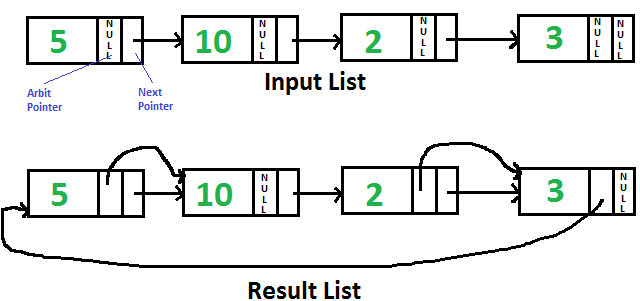GeeksforGeeks App
Open AppBrowser
Continue

# C Program For Pointing To Next Higher Value Node In A Linked List With An Arbitrary Pointer

Given singly linked list with every node having an additional “arbitrary” pointer that currently points to NULL. Need to make the “arbitrary” pointer point to the next higher value node.We strongly recommend to minimize your browser and try this yourself first

A Simple Solution is to traverse all nodes one by one, for every node, find the node which has the next greater value of the current node and change the next pointer. Time Complexity of this solution is O(n2).

An Efficient Solution works in O(nLogn) time. The idea is to use Merge Sort for linked list
1) Traverse input list and copy next pointer to arbit pointer for every node.
2) Do Merge Sort for the linked list formed by arbit pointers.

Below is the implementation of the above idea. All of the merger sort functions are taken from here. The taken functions are modified here so that they work on arbit pointers instead of next pointers.

## C

 `// C program to populate arbit pointers to``// next higher value using merge sort``#include``#include` `// Link list node``struct` `Node``{``    ``int` `data;``    ``struct` `Node* next, *arbit;``};` `// Function prototypes``struct` `Node* SortedMerge(``struct` `Node* a,``                         ``struct` `Node* b);``void` `FrontBackSplit(``struct` `Node* source,``                    ``struct` `Node** frontRef,``                    ``struct` `Node** backRef);` `/* Sorts the linked list formed by``   ``arbit pointers (does not change``   ``next pointer or data) */``void` `MergeSort(``struct` `Node** headRef)``{``    ``struct` `Node* head = *headRef;``    ``struct` `Node* a, *b;` `    ``// Base case -- length 0 or 1``    ``if` `((head == NULL) ||``        ``(head->arbit == NULL))``        ``return``;` `    ``// Split head into 'a' and 'b'``    ``// sublists``    ``FrontBackSplit(head, &a, &b);` `    ``// Recursively sort the sublists``    ``MergeSort(&a);``    ``MergeSort(&b);` `    ``// answer = merge the two sorted``    ``// lists together``    ``*headRef = SortedMerge(a, b);``}` `/* See https://www.geeksforgeeks.org/?p=3622``   ``for details of this function */``struct` `Node* SortedMerge(``struct` `Node* a,``                         ``struct` `Node* b)``{``    ``struct` `Node* result = NULL;` `    ``// Base cases``    ``if` `(a == NULL)``        ``return` `(b);``    ``else` `if` `(b==NULL)``        ``return` `(a);` `    ``// Pick either a or b, and recur``    ``if` `(a->data <= b->data)``    ``{``        ``result = a;``        ``result->arbit =``        ``SortedMerge(a->arbit, b);``    ``}``    ``else``    ``{``        ``result = b;``        ``result->arbit =``        ``SortedMerge(a, b->arbit);``    ``}` `    ``return` `(result);``}` `/* Split the nodes of the given list into``   ``front and back halves, and return the``   ``two lists using the reference parameters.``   ``If the length is odd, the extra node``   ``should go in the front list. Uses the``   ``fast/slow pointer strategy.  */``void` `FrontBackSplit(``struct` `Node* source,``                    ``struct` `Node** frontRef,``                    ``struct` `Node** backRef)``{``    ``struct` `Node* fast, *slow;` `    ``if` `(source==NULL ||``        ``source->arbit==NULL)``    ``{``        ``// length < 2 cases``        ``*frontRef = source;``        ``*backRef = NULL;``        ``return``;``    ``}` `    ``slow = source, ``    ``fast = source->arbit;` `    ``/* Advance 'fast' two nodes, and``       ``advance 'slow' one node */``    ``while` `(fast != NULL)``    ``{``        ``fast = fast->arbit;``        ``if` `(fast != NULL)``        ``{``            ``slow = slow->arbit;``            ``fast = fast->arbit;``        ``}``    ``}` `    ``/* 'slow' is before the midpoint in``        ``the list, so split it in two at``        ``that point. */``    ``*frontRef = source;``    ``*backRef = slow->arbit;``    ``slow->arbit = NULL;``}` `/* Function to insert a node at the``   ``beginning of the linked list */``void` `push(``struct` `Node** head_ref,``          ``int` `new_data)``{``    ``// Allocate node``    ``struct` `Node* new_node =``           ``(``struct` `Node*) ``malloc``(``sizeof``(``struct` `Node));` `    ``// Put in the data ``    ``new_node->data = new_data;` `    ``// Link the old list of the new node``    ``new_node->next = (*head_ref);` `    ``new_node->arbit = NULL;` `    ``// Move the head to point to``    ``// the new node``    ``(*head_ref) = new_node;``}` `// Utility function to print``// result linked list``void` `printListafter(``struct` `Node *node,``                    ``struct` `Node *anode)``{``    ``printf``(``"Traversal using Next Pointer"``);``    ``while` `(node!=NULL)``    ``{``        ``printf``(``"%d, "``, node->data);``        ``node = node->next;``    ``}` `    ``printf``(``"Traversal using Arbit Pointer"``);``    ``while` `(anode!=NULL)``    ``{``        ``printf``(``"%d, "``, anode->data);``        ``anode = anode->arbit;``    ``}``}` `// This function populates arbit pointer``// in every node to the next higher value.``// And returns pointer to the node with``// minimum value``struct` `Node* populateArbit(``struct` `Node *head)``{``    ``// Copy next pointers to arbit pointers``    ``struct` `Node *temp = head;``    ``while` `(temp != NULL)``    ``{``        ``temp->arbit = temp->next;``        ``temp = temp->next;``    ``}` `    ``// Do merge sort for arbitrary pointers``    ``MergeSort(&head);` `    ``// Return head of arbitrary pointer``    ``// linked list``    ``return` `head;``}` `// Driver code``int` `main()``{``    ``// Start with the empty list``    ``struct` `Node* head = NULL;` `    ``// Let us create the list shown above``    ``push(&head, 3);``    ``push(&head, 2);``    ``push(&head, 10);``    ``push(&head, 5);` `    ``// Sort the above created Linked List``    ``struct` `Node *ahead = populateArbit(head);` `    ``printf``(``"Result Linked List is: "``);``    ``printListafter(head, ahead);` `    ``getchar``();``    ``return` `0;``}`

Output:

```Result Linked List is:
Traversal using Next Pointer
5, 10, 2, 3,
Traversal using Arbit Pointer
2, 3, 5, 10,```

The time complexity of the populateArbit function is O(n log n), where n is the number of nodes in the linked list, due to the use of merge sort to sort the arbitrary pointers.

The space complexity is O(log n), which is the space used by the recursive stack during the merge sort algorithm.

Please refer complete article on Point to next higher value node in a linked list with an arbitrary pointer for more details!

My Personal Notes arrow_drop_up